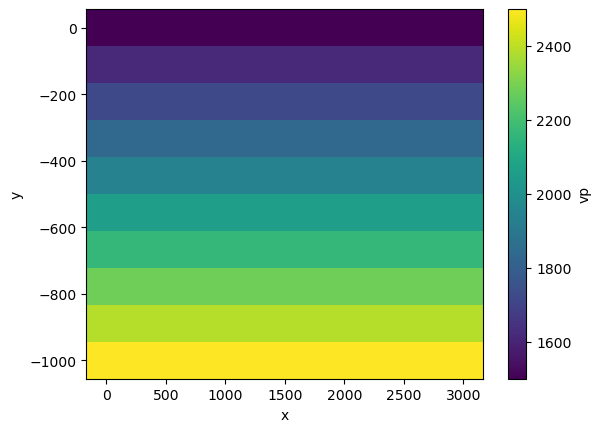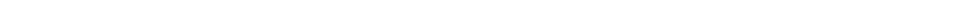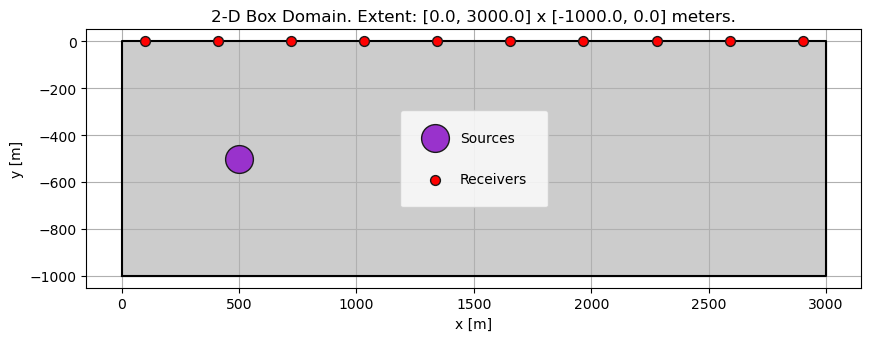Version:This tutorial is presented as Python code running inside a Jupyter Notebook, the recommended way to use Salvus. To run it yourself you can copy/type each individual cell or directly download the full notebook, including all required files.# Mapping between simulation and inversion space

It's not the destination, it's the journey
Full-waveform inversion is a non-convex problem, and the misfit landscape usually contains plenty of local minima. Therefore a suitable parameterization of the model and design of the descent method are crucial to not get trapped.
Salvus distinguishes between the model parameterizations used for the simulation and the inversion, respectively. The simulation requires a complete description of all material coefficients entering the wave equation defined on the entire domain. These are absolute quantities like velocities in m/s or density in kg/m$^3$.
However, the parameters used in the inversion can differ, for instance, by covering only a subset of the domain, a subset of material properties, different scaling relations, etc.
The link between the representation of a model in simulation space, on the one hand, and the inversion space, on the other hand, is established through the Mapping class in SalvusOpt. This gives a lot of flexibility, for instance, to use different discretizations (e.g., a coarser mesh for the inversion or an event-dependent mesh for the simulation) or model parameterizations (e.g., inverting only for a subset of the physical parameters).
This micro-tutorial presents strategies to mitigate unwanted sensitivities in the model gradients, in particular close to sources or receivers.
Copy
%matplotlib inline
%config Completer.use_jedi = False
import os
SALVUS_FLOW_SITE_NAME = os.environ.get("SITE_NAME", "local")
import matplotlib.pyplot as plt
import numpy as np
import pathlib
import time
import xarray as xr

import salvus.namespace as sn

## Project setup

To demonstrate different ways to use the mapping function, we construct a simple 2D domain with a 1D background model and a line of receivers at the surface.
nx, ny = 10, 10
x = np.linspace(0.0, 3000.0, nx)
y = np.linspace(-1000.0, 0.0, nx)
xx, yy = np.meshgrid(x, y, indexing="ij")

vp = 1500.0 - yy
rho = 1000.0 - yy

ds = xr.Dataset(
data_vars={
"vp": (["x", "y"], vp),
"rho": (["x", "y"], rho),
},
coords={"x": x, "y": y},
)
ds.vp.T.plot()
<matplotlib.collections.QuadMesh at 0x7f16539d6d50>p = sn.Project.from_volume_model(
path="project",
volume_model=sn.model.volume.cartesian.GenericModel(name="model", data=ds),
)src = sn.simple_config.source.cartesian.ScalarPoint2D(x=500.0, y=-500.0, f=1.0)
x=np.linspace(100.0, 2900.0, 10), y=0.0, fields=["phi"]
)
p.viz.nb.domain()ec = sn.EventConfiguration(
waveform_simulation_configuration=sn.WaveformSimulationConfiguration(
end_time_in_seconds=2.0
),
wavelet=sn.simple_config.stf.Ricker(center_frequency=5.0),
)
p += sn.SimulationConfiguration(
name="sim_model",
elements_per_wavelength=2,
tensor_order=4,
max_frequency_in_hertz=10.0,
model_configuration=sn.ModelConfiguration(
background_model=None, volume_models="model"
),
event_configuration=ec,
absorbing_boundaries=sn.AbsorbingBoundaryParameters(
reference_velocity=2000.0,
number_of_wavelengths=3.5,
reference_frequency=5.0,
),
)

## Synthetic data and raw gradient

Next, we create synthetic data for testing different gradient parameterizations. To keep things simple, we work without observed data, and just compute the L2 norm of the signal at the receivers. With the definition of the misfit, we have everything needed to compute gradients.
p += sn.MisfitConfiguration(
name="misfit",
observed_data=None,
misfit_function="L2_energy_no_observed_data",
)
gradients = {}
simulation_configuration="sim_model",
misfit_configuration="misfit",
wavefield_compression=sn.WavefieldCompression(
forward_wavefield_sampling_interval=1
),
events=p.events.list(),
site_name=SALVUS_FLOW_SITE_NAME,
ranks_per_job=4,
)
time.sleep(2.0)
raw_gradient = sn.UnstructuredMesh.from_h5(gradients["event"])
[2023-03-17 19:58:57,186] INFO: Creating mesh. Hang on.
[2023-03-17 19:58:57,805] INFO: Submitting job ...
[2023-03-17 19:58:58,002] INFO: Launched simulations for 1 events. Please check again to see if they are finished.
[2023-03-17 19:59:00,206] INFO: Submitting job ...
[2023-03-17 19:59:00,274] INFO: Launched adjoint simulations for 1 events. Please check again to see if they are finished.
[2023-03-17 19:59:02,323] INFO: 1 events have already been submitted. They will not be submitted again.
[2023-03-17 19:59:02,362] INFO: Some simulations are still running. Please check again to see if they are finished.
[2023-03-17 19:59:04,396] INFO: 1 events have already been submitted. They will not be submitted again.

Gradients are always computed in the simulation space first. This is the result of the adjoint simulation. We call this the raw_gradient. In the widget below, we notice that the sensitivity at the source location and - to a smaller degree - at the receiver locations has significantly higher amplitudes than everywhere else in the domain. This is the result of all energy passing through these points, which is why the waveforms are clearly most sensitive to changes at those locations. However, this clearly does not look like a reasonable model update.
raw_gradient